# awesome park simulator pjddjdjosjjs

26
3310
Share
Report

### Description

awesome park simulator pjddjdjosjjs, a project made by Massive Carriage using Tynker. Learn to code and make your own app or game in minutes.

Game

### Concepts

simple variables, basic math, visibility, advanced costume handling, variables, delays, simple messaging, miscellaneous, simple events, simple conditionals, simple loops, advanced messaging, advanced events, direction and turning, simple motion, resize actor, detect conditions, input/output, pen color, cloning, text handling, advanced motion, expert math

• #Lines:1406
• #Actors:40
• #Costumes:93
• #Scripts:193

### Text Snippets

• awesome park simulator pjddjdjosjjs
• caravan with caravan
• you must have a reception first
• jumping pillow plot
• jumping pillow happiness
• jumping pillow money

### Images

•background scene - drawing
•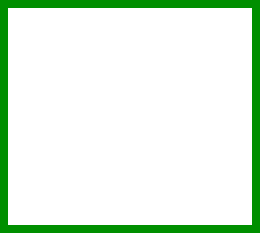plot - normal
•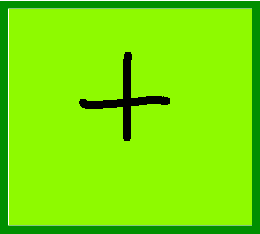•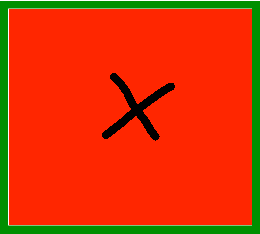•plot - caravan
•plot - caravan with caravan
•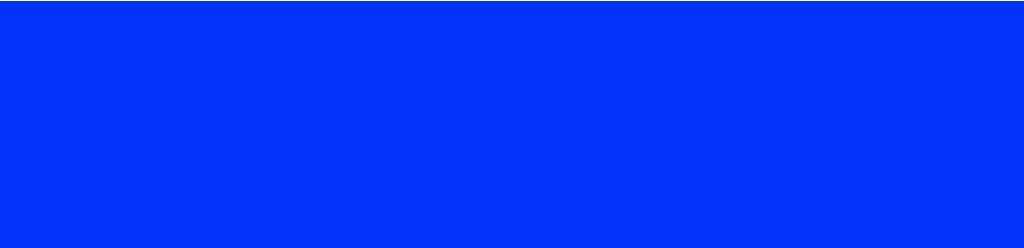shop - drawing
•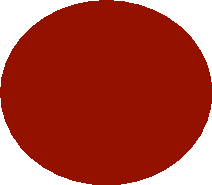shop button - drawing
•caravan plot - drawing
•plot 1 money - drawing
•drawing1 - drawing
•drawing2 - drawing
•plot 2 - normal
•••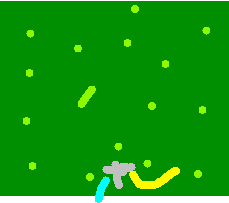plot 2 - caravan
•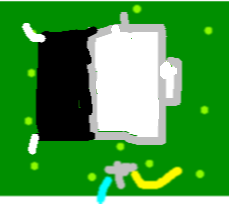plot 2 - caravan with caravan
•plot 3 - normal
•••plot 3 - caravan
•plot 3 - caravan with caravan
•plot4 - normal
•••plot4 - caravan
•plot4 - caravan with caravan
•plot5 - normal
•••plot5 - caravan
•plot5 - caravan with caravan
•plot6 - normal
•••plot6 - caravan
•plot6 - caravan with caravan
•plot 2 money - drawing
•plot 3 money - drawing
•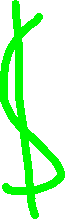plot 4 money - drawing
•plot 5 money - drawing
•plot 6 money - drawing
•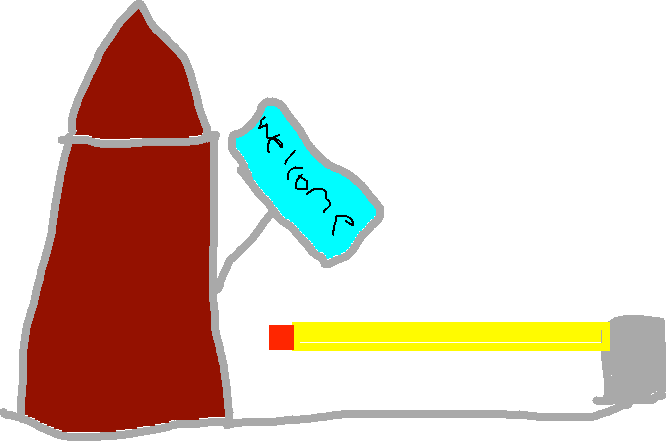reception - drawing
•reception plot - normal
•••reception plot - caravan
•reception plot - caravan with caravan
•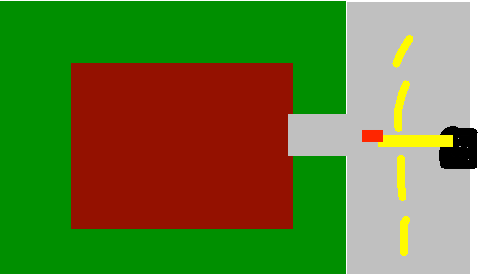reception plot - drawing
•drawing21 - drawing
•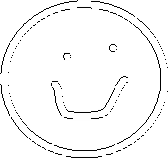drawing - drawing
•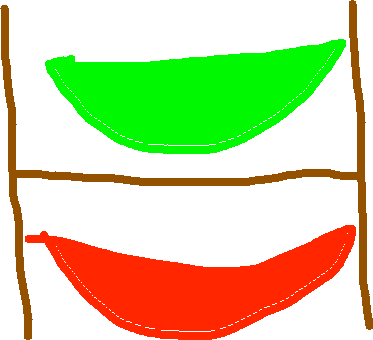kyak shop - drawing
•kayak plot - 1
•••kayak plot - caravan
•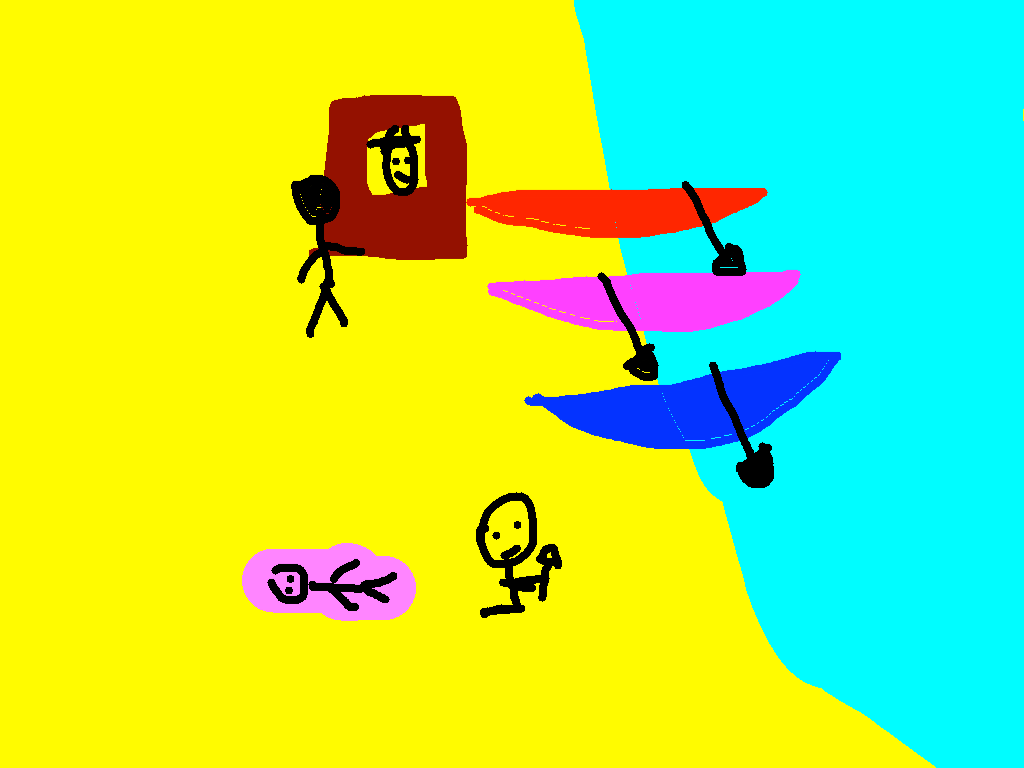kayak plot - kayak plot
•drawing22 - drawing
•kayak happenis - drawing
•jumping pillow - drawing
•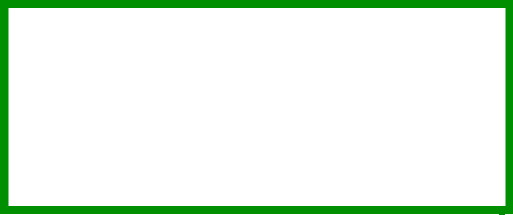jumping pillow plot - 1
••jumping pillow plot - no add
•jumping pillow plot - caravan
•jumping pillow plot - kayak plot
•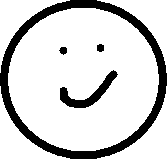jumping pillow happiness - drawing
•jumping pillow money - drawing
•plot 1 car - green car
•plot 1 car - red car
•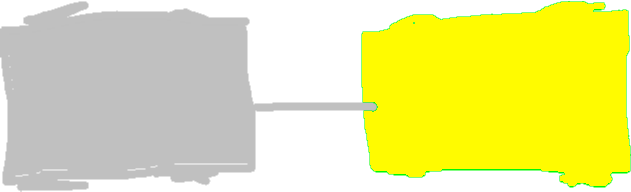plot 1 car - yellow car
•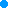drawing3 - drawing
•plot 2 car - green car
•plot 2 car - red car
•plot 2 car - yellow car
•plot 3 car - green car
•plot 3 car - red car
•plot 3 car - yellow car
•plot 4 car - green car
•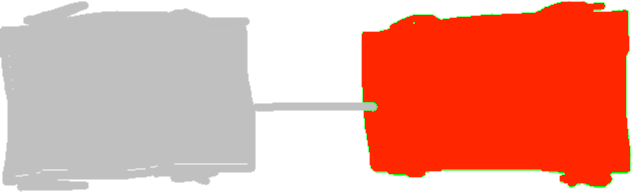plot 4 car - red car
•plot 4 car - yellow car
•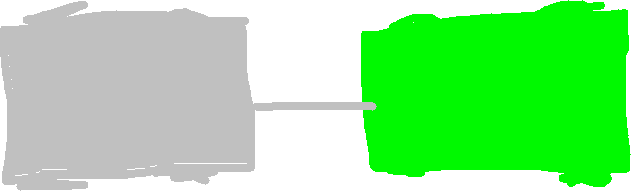plot 5 car - green car
•plot 5 car - red car
•plot 5 car - yellow car
•plot 6 car - green car
•plot 6 car - red car
•plot 6 car - yellow car
•cafe - drawing
•cafe plot - 1
••••••